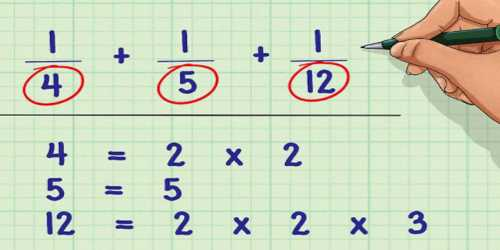Least Common Denominator

The Least Common Denominator (LCD) is the Least Common Multiple of two or more denominators. In mathematics, least common denominator is the least common multiple of the denominators of a set of fractions. It simplifies adding, subtracting, and comparing fractions. Finding the LCD is important because fractions need to have the same denominator when you are doing addition or subtraction math with fractions.

Here are the steps to follow:

• Find the Least Common Multiple of the denominators (which is called the Least Common Denominator).
• Change each fraction (using equivalent fractions) to make their denominators the same as the least common denominator.
• Then add (or subtract) the fractions, as we wish!How to find the Least Common Denominator:

• Find the Greatest Common Factor of the denominators.
• Multiply the denominators together.
• Divide the product of the denominators by the Greatest Common Factor.

Example: Find the LCD of 2/9 and 3/12

The lowest common denominator or least common denominator (abbreviated LCD) is the least common multiple of the denominators of a set of fractions. It is the smallest positive integer that is a multiple of each denominator in the set. Fractions write with fraction bar / like 3/4 .

The same fraction can be expressed in many different forms. If the ratio between numerator and denominator is the same, the fractions represent the same rational number.

The least common denominator of a set of fractions is the least number that is a multiple of all the denominators: their “least common multiple”.

Method of calculating the LCD is same as calculation least common multiple of fractions denominators.

Simple method for compute common denominator (not least at all) is multiply all denominators.

• Determine the Greatest Common Factor of 9 and 12 which is 3
• Either multiply the denominators and divide by the GCF (9*12=108, 108/3=36)
• OR – Divide one of the denominators by the GCF and multiply the quotient times the other denominator (9/3=3, 3*12=36)

How to rename fractions and use the Least Common Denominator:

• Divide the LCD by one denominator.
• Multiply the numerator times this quotient.
• Repeat the process for the other fraction(s)